xCentrifugal forceOverview

Centrifugal force can generally be any force
Force
In physics, a force is any influence that causes an object to undergo a change in speed, a change in direction, or a change in shape. In other words, a force is that which can cause an object with mass to change its velocity , i.e., to accelerate, or which can cause a flexible object to deform...

directed outward relative to some origin. More particularly, in classical mechanics
Classical mechanics
In physics, classical mechanics is one of the two major sub-fields of mechanics, which is concerned with the set of physical laws describing the motion of bodies under the action of a system of forces...

, the centrifugal force is an outward force which arises when describing the motion of objects in a rotating reference frame
Rotating reference frame
A rotating frame of reference is a special case of a non-inertial reference frame that is rotating relative to an inertial reference frame. An everyday example of a rotating reference frame is the surface of the Earth. A rotating frame of reference is a special case of a non-inertial reference...

. Because a rotating frame is an example of a non-inertial reference frame
Non-inertial reference frame
A non-inertial reference frame is a frame of reference that is under acceleration. The laws of physics in such a frame do not take on their most simple form, as required by the theory of special relativity...

, Newton's laws of motion
Newton's laws of motion
Newton's laws of motion are three physical laws that form the basis for classical mechanics. They describe the relationship between the forces acting on a body and its motion due to those forces...

do not accurately describe the dynamics within the rotating frame. However, a rotating frame can be treated as if it were an inertial frame so that Newton's laws can be used if so-called fictitious force
Fictitious force
A fictitious force, also called a pseudo force, d'Alembert force or inertial force, is an apparent force that acts on all masses in a non-inertial frame of reference, such as a rotating reference frame....

s (also known as inertial or pseudo- forces) are included in the sum of external forces on an object.Unanswered QuestionsEncyclopedia
Centrifugal force can generally be any force
Force
In physics, a force is any influence that causes an object to undergo a change in speed, a change in direction, or a change in shape. In other words, a force is that which can cause an object with mass to change its velocity , i.e., to accelerate, or which can cause a flexible object to deform...

directed outward relative to some origin. More particularly, in classical mechanics
Classical mechanics
In physics, classical mechanics is one of the two major sub-fields of mechanics, which is concerned with the set of physical laws describing the motion of bodies under the action of a system of forces...

, the centrifugal force is an outward force which arises when describing the motion of objects in a rotating reference frame
Rotating reference frame
A rotating frame of reference is a special case of a non-inertial reference frame that is rotating relative to an inertial reference frame. An everyday example of a rotating reference frame is the surface of the Earth. A rotating frame of reference is a special case of a non-inertial reference...

. Because a rotating frame is an example of a non-inertial reference frame
Non-inertial reference frame
A non-inertial reference frame is a frame of reference that is under acceleration. The laws of physics in such a frame do not take on their most simple form, as required by the theory of special relativity...

, Newton's laws of motion
Newton's laws of motion
Newton's laws of motion are three physical laws that form the basis for classical mechanics. They describe the relationship between the forces acting on a body and its motion due to those forces...

do not accurately describe the dynamics within the rotating frame. However, a rotating frame can be treated as if it were an inertial frame so that Newton's laws can be used if so-called fictitious force
Fictitious force
A fictitious force, also called a pseudo force, d'Alembert force or inertial force, is an apparent force that acts on all masses in a non-inertial frame of reference, such as a rotating reference frame....

s (also known as inertial or pseudo- forces) are included in the sum of external forces on an object. The centrifugal force is what is usually thought of as the cause for apparent outward movement like that of passengers in a vehicle turning a corner, of the weights in a centrifugal governor
Centrifugal governor
A centrifugal governor is a specific type of governor that controls the speed of an engine by regulating the amount of fuel admitted, so as to maintain a near constant speed whatever the load or fuel supply conditions...

, and of particles in a centrifuge
Centrifuge
A centrifuge is a piece of equipment, generally driven by an electric motor , that puts an object in rotation around a fixed axis, applying a force perpendicular to the axis...

. From the standpoint of an observer in an inertial frame, the effects can be explained as results of inertia
Inertia
Inertia is the resistance of any physical object to a change in its state of motion or rest, or the tendency of an object to resist any change in its motion. It is proportional to an object's mass. The principle of inertia is one of the fundamental principles of classical physics which are used to...

without invoking the centrifugal force. Centrifugal force should not be confused with centripetal force
Centripetal force
Centripetal force is a force that makes a body follow a curved path: it is always directed orthogonal to the velocity of the body, toward the instantaneous center of curvature of the path. The mathematical description was derived in 1659 by Dutch physicist Christiaan Huygens...

or the reactive centrifugal force
Reactive centrifugal force
In classical mechanics, reactive centrifugal force is the reaction paired with centripetal force. A mass undergoing circular motion constantly accelerates toward the axis of rotation. This centripetal acceleration is caused by a force exerted on the mass by some other object. In accordance with...

, both of which are real forces independent of the frame of the observer.

Analysis of motion within rotating frames can be greatly simplified by the use of the fictitious forces. By starting with an inertial frame, where Newton's laws of motion hold, and keeping track of how the time derivatives of a position vector change when transforming to a rotating reference frame, the various fictitious forces and their forms can be identified. Rotating frames and fictitious forces can often reduce the description of motion in two dimensions to a simpler description in one dimension (corresponding to a co-rotating frame). In this approach, circular motion
Circular motion
In physics, circular motion is rotation along a circular path or a circular orbit. It can be uniform, that is, with constant angular rate of rotation , or non-uniform, that is, with a changing rate of rotation. The rotation around a fixed axis of a three-dimensional body involves circular motion of...

in an inertial frame, which only requires the presence of a centripetal force, becomes the balance between the real centripetal force and the frame-determined centrifugal force
Centrifugal force
Centrifugal force can generally be any force directed outward relative to some origin. More particularly, in classical mechanics, the centrifugal force is an outward force which arises when describing the motion of objects in a rotating reference frame...

in the rotating frame where the object appears stationary. If a rotating frame is chosen so that just the angular position of an object is held fixed, more complicated motion, such as elliptical and open orbits, appears because the centripetal and centrifugal forces will not balance. The general approach however is not limited to these co-rotating frames, but can be equally applied to objects at motion in any rotating frame.

## In classical Newtonian physics

Although Newton's laws of motion hold exclusively in inertial frames, often times is far more convenient and more advantageous to describe the motion of objects within a rotating reference frame
Rotating reference frame
A rotating frame of reference is a special case of a non-inertial reference frame that is rotating relative to an inertial reference frame. An everyday example of a rotating reference frame is the surface of the Earth. A rotating frame of reference is a special case of a non-inertial reference...

. Sometimes the calculations are simpler (an example is inertial circles), and sometimes the intuitive picture coincides more closely with the rotational frame (an example is sedimentation
Sedimentation
Sedimentation is the tendency for particles in suspension to settle out of the fluid in which they are entrained, and come to rest against a barrier. This is due to their motion through the fluid in response to the forces acting on them: these forces can be due to gravity, centrifugal acceleration...

in a centrifuge). By treating the extra acceleration terms due to the rotation of the frame as if they were forces, subtracting them from the physical forces, it's possible to treat the second time derivative of position (relative to the rotating frame) as absolute acceleration. Thus the analysis using Newton's laws of motion can proceed as if the reference frame was inertial, provided the fictitious force terms are included in the sum of external forces. For example, centrifugal force is used in the FAA pilot's manual in describing turns. Other examples are such systems as planets, centrifuges, carousels, turning cars, spinning buckets, and rotating space stations.

A disadvantage of a rotating reference frame is that it can be more difficult to apply special relativity
Special relativity
Special relativity is the physical theory of measurement in an inertial frame of reference proposed in 1905 by Albert Einstein in the paper "On the Electrodynamics of Moving Bodies".It generalizes Galileo's...

(for example, from the perspective of the Earth the stars seem to traverse many light-years each day). It is possible to do so if a metric tensor
Metric tensor
In the mathematical field of differential geometry, a metric tensor is a type of function defined on a manifold which takes as input a pair of tangent vectors v and w and produces a real number g in a way that generalizes many of the familiar properties of the dot product of vectors in Euclidean...

is introduced, but the speed of light may not be constant and clocks within the frame are not synchronized.

There are three general scenarios in which this concept of a fictitious centrifugal force arises when describing motion:
1. When the motion is described relative to a rotating reference frame
Rotating reference frame
A rotating frame of reference is a special case of a non-inertial reference frame that is rotating relative to an inertial reference frame. An everyday example of a rotating reference frame is the surface of the Earth. A rotating frame of reference is a special case of a non-inertial reference...

about a fixed axis at the origin of the coordinate system. For observations made in the rotating frame, all objects appear to be under the influence of a radially outward force that is proportional to the distance from the axis of rotation and to the square of the rate of rotation (angular velocity
Angular velocity
In physics, the angular velocity is a vector quantity which specifies the angular speed of an object and the axis about which the object is rotating. The SI unit of angular velocity is radians per second, although it may be measured in other units such as degrees per second, revolutions per...

) of the frame.
2. When the motion is described using an accelerated local reference frame attached to a moving body, for example, the frame of passengers in a car as it rounds a corner. In this case, rotation is again involved, this time about the center of curvature of the path of the moving body. The first context can be seen as a special scenario within this second context in which the origin of the coordinate system and the axis of rotation are always coincident. In both, the centrifugal force is zero when the rate of rotation of the reference frame is zero, independent of the motions of objects in the frame.
3. The third context is the most general, and subsumes the first two, as well as stationary curved coordinates (e.g., polar coordinates). The centrifugal force appears when the terms for the radial component of the equation of motion are rearranged to resemble Newton's second law for one-dimensional motion. Therefore, the centrifugal force is simply the sign-reversal of the centripetal acceleration for motion along curves where the radial distance is fixed and is related to the Christoffel symbol term related to that curvature. While no rotation is necessary in this derivation, reapplying Newtonian definitions of force and acceleration to the rearranged equation necessarily implies observing the motion from a co-rotating frame of reference.

In each of these scenarios, the centrifugal force is an inertial force used for convenience and implied by a specific, non-inertial reference frame.

If objects are seen as moving within a rotating frame, this movement results in another fictitious force, the Coriolis force; and if the rate of rotation of the frame is changing, a third fictitious force, the Euler force
Euler force
In classical mechanics, the Euler acceleration , also known as azimuthal acceleration or transverse acceleration is an acceleration that appears when a non-uniformly rotating reference frame is used for analysis of motion and there is variation in the angular velocity of the reference frame's axes...

is experienced. Together, these three fictitious forces allow for the creation of correct equations of motion in a rotating reference frame.

## Derivation

For the following formalism, the rotating frame of reference is regarded as a special case of a non-inertial reference frame
Non-inertial reference frame
A non-inertial reference frame is a frame of reference that is under acceleration. The laws of physics in such a frame do not take on their most simple form, as required by the theory of special relativity...

that is rotating relative to an inertial reference frame denoted the stationary frame.

### Velocity

In a rotating frame of reference, the time derivatives of the position vector , such as velocity and acceleration vectors, of an object will differ from the time derivatives in the stationary frame according to the frame's rotation. The first time derivative evaluated within a reference frame with a coincident origin at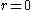but rotating with the absolute angular velocity
Angular velocity
In physics, the angular velocity is a vector quantity which specifies the angular speed of an object and the axis about which the object is rotating. The SI unit of angular velocity is radians per second, although it may be measured in other units such as degrees per second, revolutions per...

is: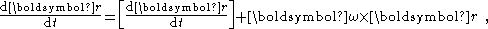where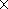denotes the vector cross product and square brackets denote evaluation in the rotating frame of reference. In other words, the apparent velocity in the rotating frame is altered by the amount of the apparent rotation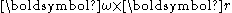at each point, which is perpendicular to both the vector from the origin and the axis of rotation and directly proportional in magnitude to each of them. The vector has magnitude equal to the rate of rotation and is directed along the axis of rotation according to the right-hand rule
Right-hand rule
In mathematics and physics, the right-hand rule is a common mnemonic for understanding notation conventions for vectors in 3 dimensions. It was invented for use in electromagnetism by British physicist John Ambrose Fleming in the late 19th century....

.

### Acceleration

Newton's law of motion for a particle of mass m written in vector form is: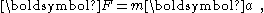where is the vector sum of the physical forces applied to the particle and is the absolute acceleration
Acceleration
In physics, acceleration is the rate of change of velocity with time. In one dimension, acceleration is the rate at which something speeds up or slows down. However, since velocity is a vector, acceleration describes the rate of change of both the magnitude and the direction of velocity. ...

(that is, acceleration in a stationary frame) of the particle, given by: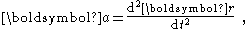where is the position vector of the particle.

By twice applying the transformation above from the stationary to the rotating frame, the absolute acceleration of the particle can be written as: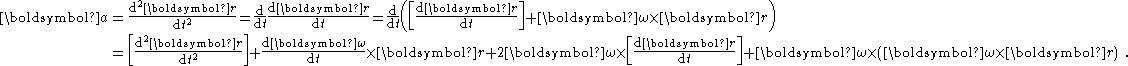### Force

The apparent acceleration in the rotating frame is [d2r/dt2]. An observer unaware of the rotation would expect this to be zero in the absence of outside forces. However Newton's laws of motion apply only in the stationary frame and describe dynamics in terms of the absolute acceleration d2r/dt2. Therefore the observer perceives the extra terms as contributions due to fictitious forces. These terms in the apparent acceleration are independent of mass; so it appears that each of these fictitious forces, like gravity, pulls on an object in proportion to its mass. When these forces are added, the equation of motion has the form: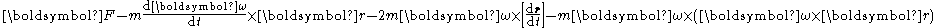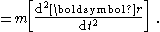From the perspective of the rotating frame, the additional force terms are experienced just like the real external forces and contribute to the apparent acceleration. The additional terms on the force side of the equation can be recognized as, reading from left to right, the Euler force
Euler force
In classical mechanics, the Euler acceleration , also known as azimuthal acceleration or transverse acceleration is an acceleration that appears when a non-uniformly rotating reference frame is used for analysis of motion and there is variation in the angular velocity of the reference frame's axes...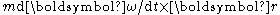, the Coriolis force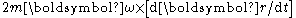, and the centrifugal force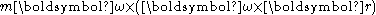, respectively. Unlike the other two fictitious forces, the centrifugal force always points radially outward from the axis of rotation of the rotating frame, with magnitude , and unlike the Coriolis force in particular, it is independent of the motion of the particle in the rotating frame. As expected, for a non-rotating inertial frame of reference
Inertial frame of reference
In physics, an inertial frame of reference is a frame of reference that describes time homogeneously and space homogeneously, isotropically, and in a time-independent manner.All inertial frames are in a state of constant, rectilinear motion with respect to one another; they are not...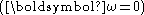the centrifugal force and all other fictitious forces disappear.

## Absolute rotation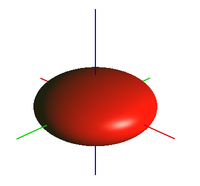Three scenarios were suggested by Newton to answer the question of whether the absolute rotation of a local frame can be detected; that is, if an observer can decide whether an observed object is rotating or if the observer is rotating.
• The shape of the surface of water rotating in a bucket. Centrifugal force will force the surface of the water to be concave.
• The tension in a string joining two spheres rotating
Rotating spheres
Isaac Newton's rotating spheres argument attempts to demonstrate that true rotational motion can be defined by observing the tension in the string joining two identical spheres...

about their center of mass. The tension in the string will be proportional to the centrifugal force on each sphere as it rotates around the common center of mass.

In these scenarios, the effects attributed to centrifugal force are only observed in the local frame (the frame in which the object is stationary) if the object is undergoing absolute rotation relative to an inertial frame. By contrast, in an inertial frame, the observed effects arise as a consequence of the inertia and the known forces without the need to introduce a centrifugal force. Based on this argument, the privileged frame, wherein the laws of physics take on the simplest form, is a stationary frame in which no fictitious forces need to be invoked.

Within this view of physics, any other phenomenon that is usually attributed to centrifugal force can be used to identify absolute rotation. For example, the oblateness of a sphere of freely flowing material is often explained in terms of centrifugal force. The oblate spheroid shape reflects, following Clairaut's theorem
Clairaut's theorem
Clairaut's theorem, published in 1743 by Alexis Claude Clairaut in his Théorie de la figure de la terre, tirée des principes de l'hydrostatique, synthesized physical and geodetic evidence that the Earth is an oblate rotational ellipsoid. It is a general mathematical law applying to spheroids of...

, the balance between containment by gravitational attraction and dispersal by centrifugal force. That the Earth is itself an oblate spheroid is taken as one of the evidences for its absolute rotation. The idea is that during a daily rotation, a portion of the Earth at the equator traverses a circle around the axis of rotation larger than a portion near the pole, and this more rapid circular motion means the centrifugal force upon the rotating portion near the equator is larger, causing the material near the equator to bulge outward.

## Examples

Below several examples illustrate both the stationary and rotating frames of reference, and the role of centrifugal force and its relation to Coriolis force in rotating frameworks. For more examples see Fictitious force, rotating bucket and rotating spheres.

### Dropping ball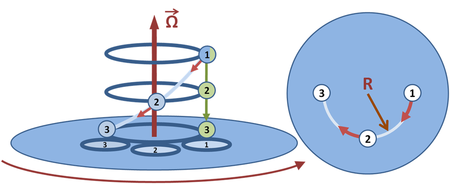An example of straight-line motion as seen in a stationary frame is a ball that steadily drops at a constant rate parallel to the axis of rotation. From a stationary frame of reference it moves in a straight line, but from the rotating frame it moves in a spiral. The projection of the spiral motion in a rotating horizontal plane is shown at the right of the figure. Because the projected horizontal motion in the rotating frame is a circular motion, the ball's motion requires an inward centripetal force, provided in this case by a fictitious force that produces the apparent spiral motion. This force is the sum of an outward centrifugal force and an inward Coriolis force. The Coriolis force overcompensates the centrifugal force by exactly the required amount to provide the necessary centripetal force to achieve circular motion.

### Banked turn

Riding a car around a curve, we take a personal view that we are at rest in the car, and should be undisturbed in our seats. Nonetheless, we feel sideways force applied to us from the seats and doors and a need to lean to one side. To explain the situation, we propose a centrifugal force that is acting upon us and must be combated. Interestingly, we find this discomfort is reduced when the curve is banked, tipping the car inward toward the center of the curve.

A different point of view is that of the highway designer. The designer views the car as executing curved motion and therefore requiring an inward centripetal force to impel the car around the turn. By banking the curve, the force exerted upon the car in a direction normal to the road surface has a horizontal component that provides this centripetal force. That means the car tires no longer need to apply a sideways force to the car, but only a force perpendicular to the road. By choosing the angle of bank to match the car's speed around the curve, the car seat transmits only a perpendicular force to the passengers, and the passengers no longer feel a need to lean nor feel a sideways push by the car seats or doors.

### Earth

A calculation for Earth
Earth
Earth is the third planet from the Sun, and the densest and fifth-largest of the eight planets in the Solar System. It is also the largest of the Solar System's four terrestrial planets...

at the equator (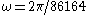seconds,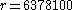meters) shows that an object experiences a centrifugal force equal to approximately 1/289 of standard gravity
Standard gravity
Standard gravity, or standard acceleration due to free fall, usually denoted by g0 or gn, is the nominal acceleration of an object in a vacuum near the surface of the Earth. It is defined as precisely , or about...

. Because centrifugal force increases according to the square of, one would expect gravity to be cancelled for an object travelling 17 times faster than the Earth's rotation, and in fact satellites in low orbit at the equator complete 17 full orbit
Orbit
In physics, an orbit is the gravitationally curved path of an object around a point in space, for example the orbit of a planet around the center of a star system, such as the Solar System...

s in one day.

Gravity diminishes according to the inverse square of distance, but centrifugal force increases in direct proportion to the distance. Thus a circular geosynchronous orbit
Geosynchronous orbit
A geosynchronous orbit is an orbit around the Earth with an orbital period that matches the Earth's sidereal rotation period...

has a radius of 42164 km; 42164/6378.1 = 6.61, the cube root of 289.

### Planetary motion

Centrifugal force arises in the analysis of orbital motion and, more generally, of motion in a central-force field: in the case of a two-body problem
Two-body problem
In classical mechanics, the two-body problem is to determine the motion of two point particles that interact only with each other. Common examples include a satellite orbiting a planet, a planet orbiting a star, two stars orbiting each other , and a classical electron orbiting an atomic nucleus In...

, it is easy to convert to an equivalent one-body problem with force directed to or from an origin, and motion in a plane, so we consider only that.

The symmetry of a central force lends itself to a description in polar coordinates. The dynamics of a mass, m, expressed using Newton's second law of motion
Newton's laws of motion
Newton's laws of motion are three physical laws that form the basis for classical mechanics. They describe the relationship between the forces acting on a body and its motion due to those forces...

(F = ma), becomes in polar coordinates: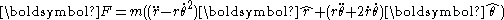where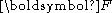is the force accelerating the object and the "hat" variables are unit direction vectors (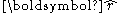points in the centrifugal or outward direction, and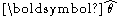is orthogonal to it).

In the case of a central force, relative to the origin of the polar coordinate system,can be replaced by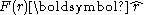, meaning the entire force is the component in the radial direction. An inward force of gravity would therefore correspond to a negative-valued F(r).

The components of F =
ma along the radial direction therefore reduce to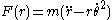in which the term proportional to the square of the rate of rotation appears on the acceleration side as a "centripetal acceleration", that is, a negative acceleration term in thedirection. In the special case of a planet in circular orbit around its star, for example, where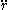is zero, the centripetal acceleration alone is the entire acceleration of the planet, curving its path toward the sun under the force of gravity, the negative F(r).

As pointed out by Taylor, for example, it is sometimes convenient to work in a co-rotating frame, that is, one rotating with the object so that the angular rate of the frame,, equals the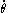of the object in the stationary frame. In such a frame, the observedis zero andalone is treated as the acceleration: so in the equation of motion, the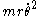term is "reincarnated on the force side of the equation (with opposite signs, of course) as the centrifugal force 2r in the radial equation": The "reincarnation" on the force side of the equation is necessary because, without this force term, observers in the rotating frame would find they could not predict the motion correctly. They would have an incorrect radial equation: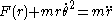where theterm is known as the centrifugal force. The centrifugal force term in this equation is called a "fictitious force", "apparent force", or "pseudo force", as its value varies with the rate of rotation of the frame of reference
Frame of reference
A frame of reference in physics, may refer to a coordinate system or set of axes within which to measure the position, orientation, and other properties of objects in it, or it may refer to an observational reference frame tied to the state of motion of an observer.It may also refer to both an...

. When the centrifugal force term is expressed in terms of parameters of the rotating frame, replacingwith, it can be seen that it is the same centrifugal force previously derived for rotating reference frames.

Because of the absence of a net force in the azimuthal direction, conservation of angular momentum allows the radial component of this equation to be expressed solely with respect to the radial coordinate, r, and the angular momentum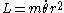, yielding the radial equation (a "fictitious one-dimensional problem" with only an r dimension):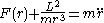.

The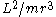term is again the centrifugal force, a force component induced by the rotating frame of reference. The equations of motion for r that result from this equation for the rotating 2D frame are the same that would arise from a particle in a fictitious one-dimensional scenario under the influence of the force in the equation above. If F(r) represents gravity, it is a negative term proportional to 1/r2, so the net acceleration in r in the rotating frame depends on a difference of reciprocal square and reciprocal cube terms, which are in balance in a circular orbit but otherwise typically not. This equation of motion is similar to one originally proposed by Leibniz. Given r, the rate of rotation is easy to infer from the constant angular momentum L, so a 2D solution can be easily reconstructed from a 1D solution of this equation.

When the angular velocity of this co-rotating frame is not constant, that is, for non-circular orbits, other fictitious forces—the Coriolis force and the Euler force
Euler force
In classical mechanics, the Euler acceleration , also known as azimuthal acceleration or transverse acceleration is an acceleration that appears when a non-uniformly rotating reference frame is used for analysis of motion and there is variation in the angular velocity of the reference frame's axes...

—will arise, but can be ignored since they will cancel each other, yielding a net zero acceleration transverse to the moving radial vector, as required by the starting assumption that the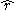vector co-rotates with the planet. In the special case of circular orbits, in order for the radial distance to remain constant the outward centrifugal force must cancel the inward force of gravity; for other orbit shapes, these forces will not cancel, so r will not be constant.

## History

Concepts of centripetal and centrifugal force played a key early role in establishing the set of inertial frames of reference and the significance of fictitious forces, even aiding in the development of general relativity
General relativity
General relativity or the general theory of relativity is the geometric theory of gravitation published by Albert Einstein in 1916. It is the current description of gravitation in modern physics...

in which gravity itself becomes a fictitious force.

## Applications

The operations of numerous common rotating mechanical systems are most easily conceptualized in terms of centrifugal force. For example:
• A centrifugal governor
Centrifugal governor
A centrifugal governor is a specific type of governor that controls the speed of an engine by regulating the amount of fuel admitted, so as to maintain a near constant speed whatever the load or fuel supply conditions...

regulates the speed of an engine by using spinning masses that move radially, adjusting the throttle
Throttle
A throttle is the mechanism by which the flow of a fluid is managed by constriction or obstruction. An engine's power can be increased or decreased by the restriction of inlet gases , but usually decreased. The term throttle has come to refer, informally and incorrectly, to any mechanism by which...

, as the engine changes speed. In the reference frame of the spinning masses, centrifugal force causes the radial movement.
• A centrifugal clutch
Centrifugal clutch
A centrifugal clutch is a clutch that uses centrifugal force to connect two concentric shafts, with the driving shaft nested inside the driven shaft....

is used in small engine-powered devices such as chain saws, go-karts and model helicopters. It allows the engine to start and idle without driving the device but automatically and smoothly engages the drive as the engine speed rises. Inertial drum brake ascenders used in rock climbing
Rock climbing
Rock climbing also lightly called 'The Gravity Game', is a sport in which participants climb up, down or across natural rock formations or artificial rock walls. The goal is to reach the summit of a formation or the endpoint of a pre-defined route without falling...

and the inertia reels used in many automobile seat belts operate on the same principle.
• Centrifugal forces can be used to generate artificial gravity
Artificial gravity
Artificial gravity is the varying of apparent gravity via artificial means, particularly in space, but also on the Earth...

, as in proposed designs for rotating space stations. The Mars Gravity Biosatellite
Mars Gravity Biosatellite
The Mars Gravity Biosatellite was a project initiated in 2001 by the Mars Society and volunteer students and staff of MIT to build a spacecraft to study the effects of Mars-level gravity on mammals. On 24 June 2009, a status report was released declaring the end of this program, due to lack of...

will study the effects of Mars
Mars
Mars is the fourth planet from the Sun in the Solar System. The planet is named after the Roman god of war, Mars. It is often described as the "Red Planet", as the iron oxide prevalent on its surface gives it a reddish appearance...

-level gravity on mice with gravity simulated in this way.
• Spin casting
Spin casting
Spin casting, also known as centrifugal rubber mold casting , is a method of utilizing centrifugal force to produce castings from a rubber mold. Typically, a disc-shaped mold is spun along its central axis at a set speed. The casting material, usually molten metal or liquid thermoset plastic is...

and centrifugal casting
Centrifugal casting (industrial)
Centrifugal casting or rotocasting is a casting technique that is typically used to cast thin-walled cylinders. It is noted for the high quality of the results attainable, particularly for precise control of their metallurgy and crystal structure...

are production methods that uses centrifugal force to disperse liquid metal or plastic throughout the negative space of a mold.
• Centrifuge
Centrifuge
A centrifuge is a piece of equipment, generally driven by an electric motor , that puts an object in rotation around a fixed axis, applying a force perpendicular to the axis...

s are used in science and industry to separate substances. In the reference frame spinning with the centrifuge, the centrifugal force induces a hydrostatic pressure gradient in fluid-filled tubes oriented perpendicular to the axis of rotation, giving rise to large buoyant forces which push low-density particles inward. Elements or particles denser than the fluid move outward under the influence of the centrifugal force. This is effectively Archimedes' principle
Archimedes' principle
Archimedes' principle relates buoyancy to displacement. It is named after its discoverer, Archimedes of Syracuse.-Principle:Archimedes' treatise On floating bodies, proposition 5, states that...

as generated by centrifugal force as opposed to being generated by gravity.
• Some amusement ride
Amusement ride
Amusement rides are large mechanical devices that move people to create enjoyment. They are frequently found at amusement parks, traveling carnivals, and funfairs.-Notable types:*Afterburner*Ali Baba*Balloon Race*Booster...

s make use of centrifugal forces. For instance, a Gravitron
Gravitron
The Gravitron is an amusement ride, most commonly found as a portable ride at fairs and carnivals. The Gravitron first appeared at Morey's Piers in 1983 and quickly became a fixture at amusement parks in many countries. It is a modification of an earlier ride called the Rotor...

's spin forces riders against a wall and allows riders to be elevated above the machine's floor in defiance of Earth's gravity.

Nevertheless, all of these systems can also be described without requiring the concept of centrifugal force, in terms of motions and forces in a stationary frame, at the cost of taking somewhat more care in the consideration of forces and motions within the system.

## See also

• Equivalence principle
Equivalence principle
In the physics of general relativity, the equivalence principle is any of several related concepts dealing with the equivalence of gravitational and inertial mass, and to Albert Einstein's assertion that the gravitational "force" as experienced locally while standing on a massive body is actually...

• Folk physics
• Lamm equation
Lamm equation
The Lamm equation describes the sedimentation and diffusion of a solute under ultracentrifugation in traditional sector-shaped cells. It was named after Ole Lamm, later professor of physical chemistry at the Royal Institute of Technology, who derived it during his Ph.D...

• Lagrangian point
Lagrangian point
The Lagrangian points are the five positions in an orbital configuration where a small object affected only by gravity can theoretically be stationary relative to two larger objects...

## External links

The source of this article is wikipedia, the free encyclopedia.  The text of this article is licensed under the GFDL.